Test: Control Systems - 2

# Test: Control Systems - 2

Test Description

## 25 Questions MCQ Test Electrical Engineering SSC JE (Technical) | Test: Control Systems - 2

Test: Control Systems - 2 for Electrical Engineering (EE) 2022 is part of Electrical Engineering SSC JE (Technical) preparation. The Test: Control Systems - 2 questions and answers have been prepared according to the Electrical Engineering (EE) exam syllabus.The Test: Control Systems - 2 MCQs are made for Electrical Engineering (EE) 2022 Exam. Find important definitions, questions, notes, meanings, examples, exercises, MCQs and online tests for Test: Control Systems - 2 below.
Solutions of Test: Control Systems - 2 questions in English are available as part of our Electrical Engineering SSC JE (Technical) for Electrical Engineering (EE) & Test: Control Systems - 2 solutions in Hindi for Electrical Engineering SSC JE (Technical) course. Download more important topics, notes, lectures and mock test series for Electrical Engineering (EE) Exam by signing up for free. Attempt Test: Control Systems - 2 | 25 questions in 50 minutes | Mock test for Electrical Engineering (EE) preparation | Free important questions MCQ to study Electrical Engineering SSC JE (Technical) for Electrical Engineering (EE) Exam | Download free PDF with solutions
 1 Crore+ students have signed up on EduRev. Have you?
Test: Control Systems - 2 - Question 1

### The transform function of a system is the Laplace transform of

Detailed Solution for Test: Control Systems - 2 - Question 1

The impulse response of a system is given by the transfer function.

If the transfer function of a system is given by H(s), then the impulse response of a system is given by h(t) where h(t) is the inverse Laplace Transform of H(s).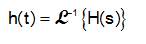Test: Control Systems - 2 - Question 2

### The transfer function is defined as

Test: Control Systems - 2 - Question 3

### What is the characteristic of a good control system ?

Test: Control Systems - 2 - Question 4

The transfer function of a linear system is the

Test: Control Systems - 2 - Question 5

If the open-loop transfer function is a ratio of a numerator polynomial of degree 'm' and a denominator polynomial of degree 'n' then the integer (n-m) represents the number of

Test: Control Systems - 2 - Question 6

Despite the presence of negative feedback, control system still have problems of instability because the

Test: Control Systems - 2 - Question 7

Consider the following statements in connection with the feedback of control systems:
1. Feedback can improve stability or be harmful to stability if it is not properly applied.
2. Feedback can always improve stability.
3. In many situations the feedback can reduce the effect of noise and disturbance on system performance.
4. In general the sensitivity of the system gain of a feedback system to a parameter variatio
depends on, where the parameter is located.
Which of the above statements are correct ?

Test: Control Systems - 2 - Question 8

Consider the following statements:
1. The effect of feedback is to reduce the system error.
2. Feedback increases the gain of the system in one frequency range but decreases in another.
3. Feedback can cause a system that is originally stable to become unstable.
Which of these statements are correct ?

Test: Control Systems - 2 - Question 9

Consider the following statements: Feedback in control system can be used
1. to reduce the sensitivity of the system to parameter variations and disturbances.
2. to change time constant of the system.
3. to increase loop gain of the system
Which of the statement given above are correct ?

Test: Control Systems - 2 - Question 10

With negative feedback in a closed loop control system, the system sensitivity to paramete variations

Test: Control Systems - 2 - Question 11

In a control system, the use of negative feedback

Test: Control Systems - 2 - Question 12

The transfer function of a system is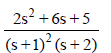The characteristic equation of the system is

Test: Control Systems - 2 - Question 13

Which one of the following identity is correct one ?

Test: Control Systems - 2 - Question 14

The transfer function from d(s) to y(s) is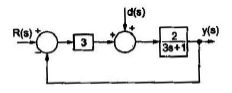Test: Control Systems - 2 - Question 15

For what value of K, are the two block diagrams as shown below equivalent ?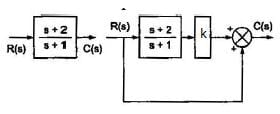Test: Control Systems - 2 - Question 16

In the signal flow graph of figure, y/x equals.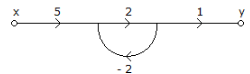Detailed Solution for Test: Control Systems - 2 - Question 16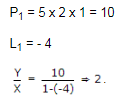Test: Control Systems - 2 - Question 17

For the signal flow diagram shown in the given figure, the transmittance between x2 and x1 is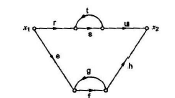Test: Control Systems - 2 - Question 18

When a human being tries to approach an object, his brain acts as?​

Test: Control Systems - 2 - Question 19

Consider the loop transfer function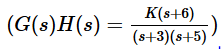In the roots locus diagram the controid will be located at

Test: Control Systems - 2 - Question 20

The number of roots of the polynomial, s7 + s6 + 7s5 + 14s4 + 31s3 + 73s2 + 25s + 200, in the open left half of the complex plane is

Detailed Solution for Test: Control Systems - 2 - Question 20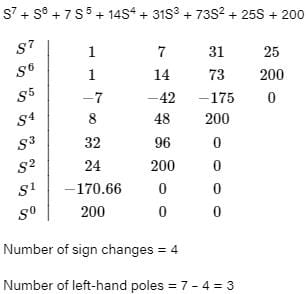Test: Control Systems - 2 - Question 21

The gain at the breakaway point of the root locus of a unity feedback system with open loop transfer function

G(s)=Ks/{(s−1)(s−4)} is

Detailed Solution for Test: Control Systems - 2 - Question 21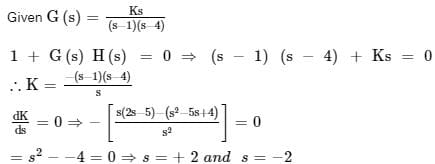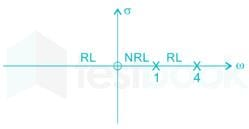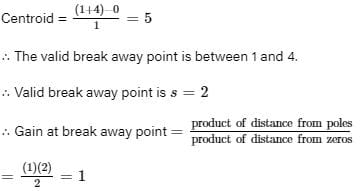Test: Control Systems - 2 - Question 22

First column elements of the Routh's tabulation are 3, 5 -3/4, 1/2, 2. It means that there

Test: Control Systems - 2 - Question 23

The response c(t) of a system is described by te differential equation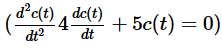The system response is

Test: Control Systems - 2 - Question 24

The polar plot of a transfer function passes through the critical point (–1.0). The gain margin is

Test: Control Systems - 2 - Question 25

If the transfer function of a phase lead compensator is (s+ a)/ (s+b) and that of a lag compensator is (s + p)/(s+q), then Which one of the following sets of condition must be satisfied ?

## Electrical Engineering SSC JE (Technical)

2 videos|65 docs|37 tests
 Use Code STAYHOME200 and get INR 200 additional OFF Use Coupon Code
Information about Test: Control Systems - 2 Page
In this test you can find the Exam questions for Test: Control Systems - 2 solved & explained in the simplest way possible. Besides giving Questions and answers for Test: Control Systems - 2, EduRev gives you an ample number of Online tests for practice

## Electrical Engineering SSC JE (Technical)

2 videos|65 docs|37 tests